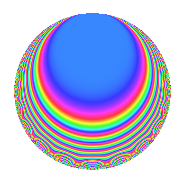# Properties

 Label 1575.1.cpLevel $1575$ Weight $1$ Character orbit 1575.cp Rep. character $\chi_{1575}(193,\cdot)$ Character field $\Q(\zeta_{12})$ Dimension $8$ Newform subspaces $1$ Sturm bound $240$ Trace bound $0$

# Related objects

## Defining parameters

 Level: $$N$$ $$=$$ $$1575 = 3^{2} \cdot 5^{2} \cdot 7$$ Weight: $$k$$ $$=$$ $$1$$ Character orbit: $$[\chi]$$ $$=$$ 1575.cp (of order $$12$$ and degree $$4$$) Character conductor: $$\operatorname{cond}(\chi)$$ $$=$$ $$315$$ Character field: $$\Q(\zeta_{12})$$ Newform subspaces: $$1$$ Sturm bound: $$240$$ Trace bound: $$0$$

## Dimensions

The following table gives the dimensions of various subspaces of $$M_{1}(1575, [\chi])$$.

Total New Old
Modular forms 56 24 32
Cusp forms 8 8 0
Eisenstein series 48 16 32

The following table gives the dimensions of subspaces with specified projective image type.

$$D_n$$ $$A_4$$ $$S_4$$ $$A_5$$
Dimension 0 8 0 0

## Trace form

 $$8q + 4q^{6} + O(q^{10})$$ $$8q + 4q^{6} - 4q^{16} + 8q^{21} - 4q^{26} + 4q^{31} - 4q^{41} + 4q^{51} - 8q^{56} - 4q^{61} - 16q^{71} - 8q^{81} - 8q^{86} + 4q^{91} + O(q^{100})$$

## Decomposition of $$S_{1}^{\mathrm{new}}(1575, [\chi])$$ into newform subspaces

Label Dim. $$A$$ Field Image CM RM Traces $q$-expansion
$$a_2$$ $$a_3$$ $$a_5$$ $$a_7$$
1575.1.cp.a $$8$$ $$0.786$$ $$\Q(\zeta_{24})$$ $$A_{4}$$ None None $$0$$ $$0$$ $$0$$ $$0$$ $$q-\zeta_{24}^{11}q^{2}-\zeta_{24}^{9}q^{3}-\zeta_{24}^{8}q^{6}+\cdots$$